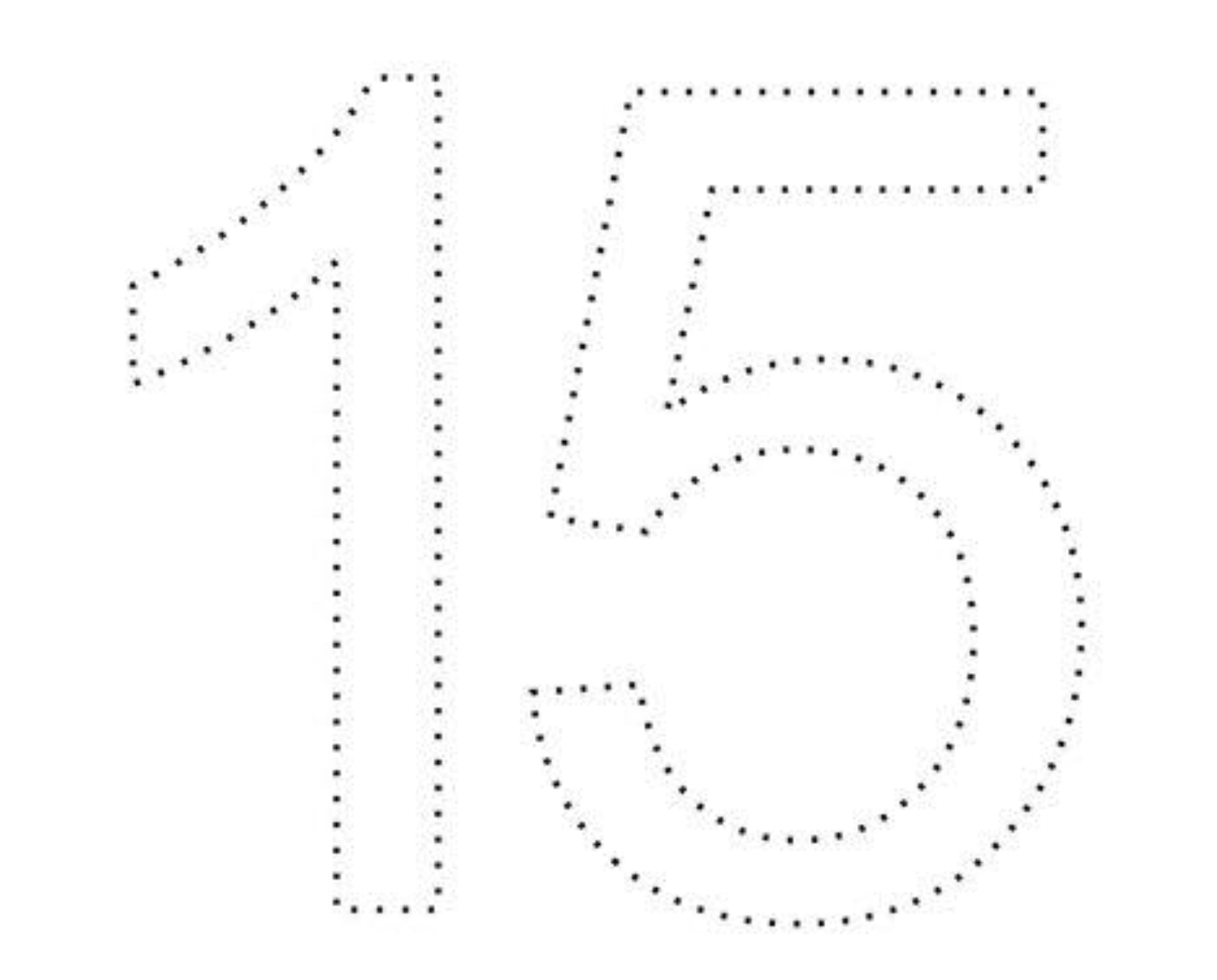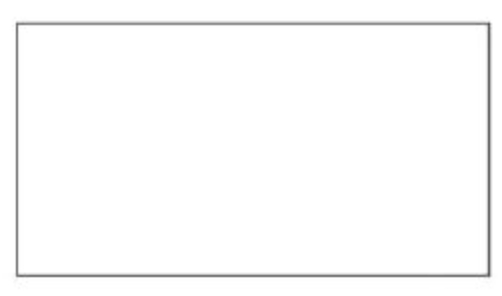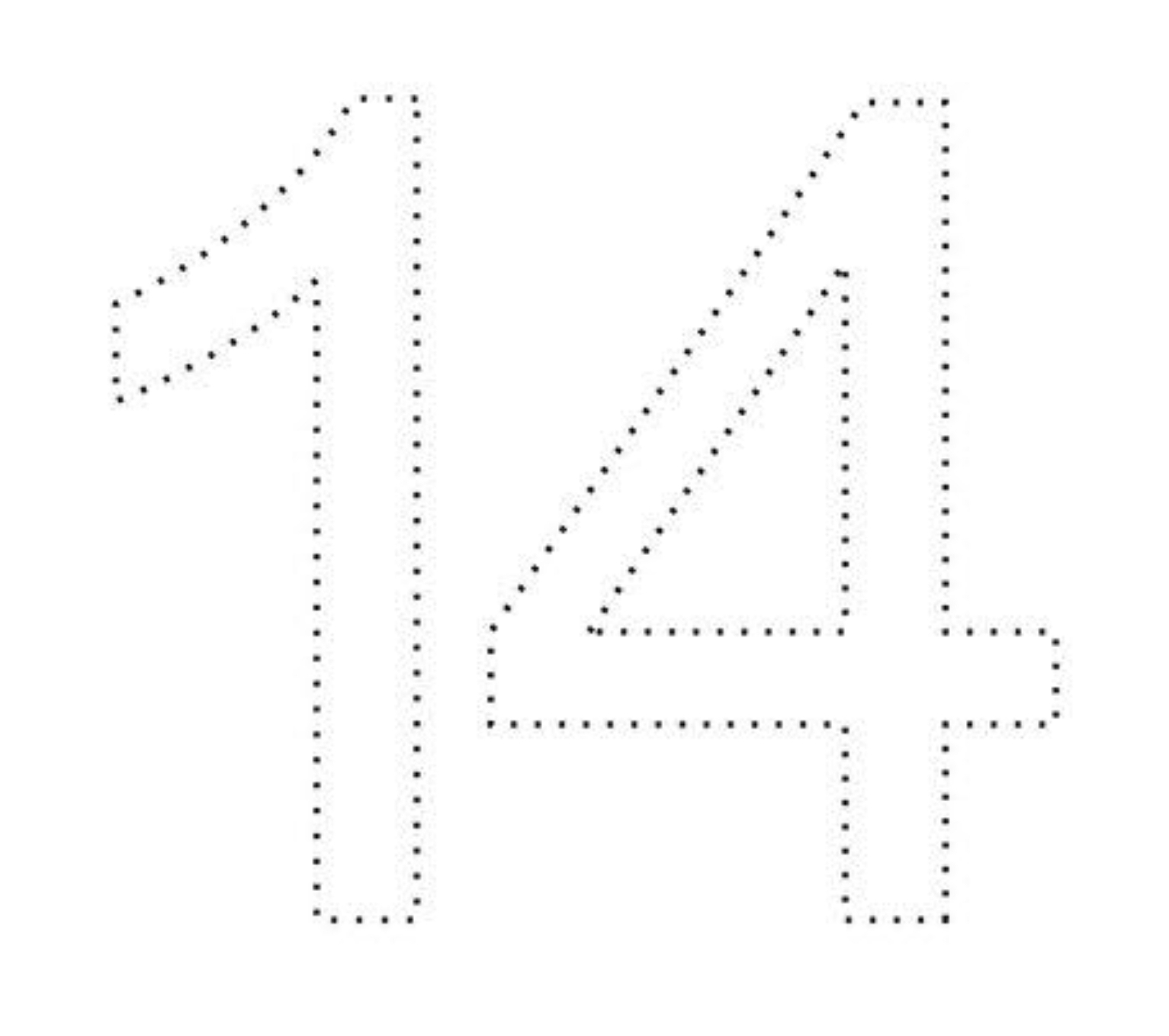# Third Term Examination Number Work Nursery 1 (Age 3) – Exam Questions

### SECTION A

1. Write 1 – 20.

2. Count and write.

### ✏️✏️✏️✏️ =

3. Read from 1 – 20.

### 13 14 15 16 17 18 19 20

4. Match same number.

### 9             3

5. Recite 1 – 30.

6. Count on strokes

6 =

4 =

12 =

7. Circle numbers 7, 9, 13 , 15 and 19.

### 13 14 15 16 17 18 19 20

8. Colour the shapes.

9. Name the following shapes[a] Rectangle

[b] Triangle[a] Rectangle

[b] Square

10. Identify and colour the numbers.